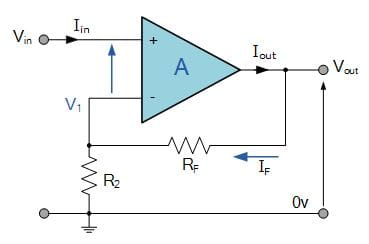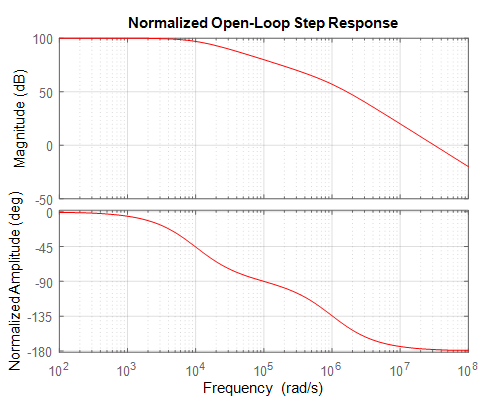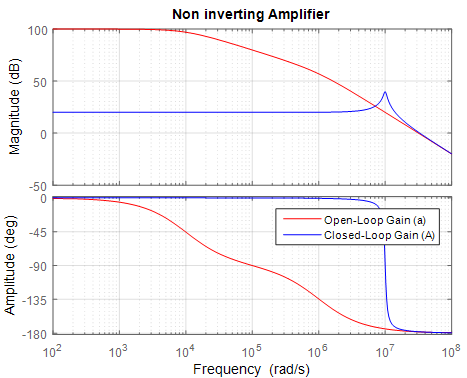Matlab Code for Designing of Non-Inverting Amplifier - Matlab Simulink for E & T

## Tuesday, July 7, 2020Non-Inverting Amplifier Using Matlab

# Non-Inverting Amplifier

In matlab simulink we can design the non-inverting amplifier easily but if we want to design by MATLAB Program then need non inverting amplifier transfer. here we discuss only how to design  non-inverting amplifier.

so firstly know about the non-inverting amplifier then derive the transfer function then coding in matlab and analysis -

# This emerges from the way that the gain of the amplifier is extremely high. On the off chance that the yield of the system remains within the supply rails of the amplifier, then the yield voltage divided by the gain.

For best laptop for Matlab software-  https://amzn.to/39QeZ9d

Camera- https://amzn.to/39KBi02

Professional tripod- https://amzn.to/39Jg96w# The voltage gain can be calculated by applying KCL at the inverting node.

Voltage gain (A) = Vout / Vin = (1+ RRin)

Matlab Code / Program -

The Design Non-Inverting Amplifier Using Matlab follow some steps -

Ø open the editor window in matlab (shortcut key CTRL+N)

Ø write down the following matlab code in editor window

clc ;

clear ;

close all ;

a0 = 1e5 ;

w1 = 1e4 ;

w2 = 1e6 ;

s = tf('s') ;

a = a0/(1+s/w1)/(1+s/w2)

figure ,

h = bodeplot(a,'r') ;

title(' Normalized Open-Loop Step Response ') ;

ylabel(' Normalized Amplitude ') ;

grid

A0 = 10 ;

b = 1 / A0 ;         % approximation for ab > > 1

R1 = 10000 ;

R2 = R1*(1/b-1) ;

A = feedback(a,b) ;

figure ,

bode( a ,' r ' , A , ' b ' ) ;

grid

After complete write code then save the code and run

for run press F5 key and output show in command window

Output Result-

a =

1e15

----------------------

s^2 + 1.01e06 s + 1e10

Continuous-time transfer function.fig. 1 - Normalized Open-Loop Step Responsefig. 2 - Normalized close-Loop with feedback Step Response

the figure 1 show the Step Response of the Normalized Open-Loop system (non aplifier) and figure 2 show the Step Response of the Normalized close-Loop with feedback

Related Post –

# 1st order R-C circuits using matlab

For more matlab code and program

Please Like, Comment, follow our blog for new updates and Share and email subscribe for latest update

! THANK YOU !